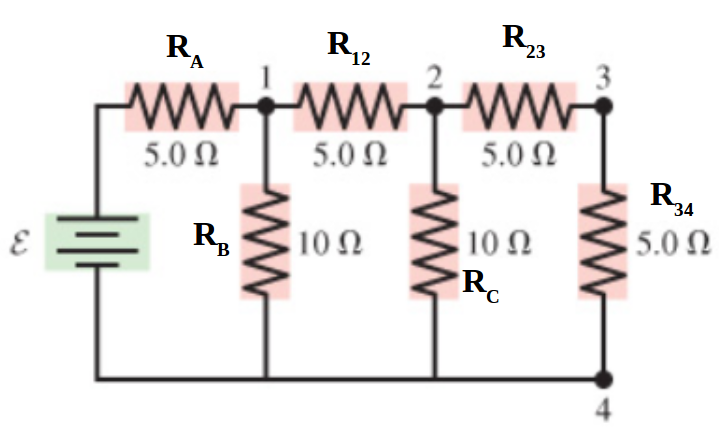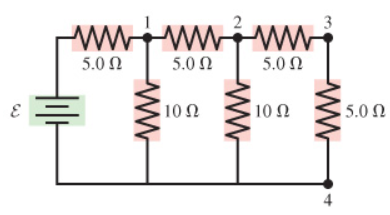# Problem: Consider the potential difference between pairs of points in the figure. Suppose that ε = 7.5 V .(a) What is the magnitude of the potential difference ΔV14 ?(b) What is the magnitude of the potential difference ΔV24 ?(c) What is the magnitude of the potential difference ΔV34 ?Express your answers to two significant figures and include the appropriate units.

###### FREE Expert Solution

Solution steps:

1. Find equivalent resistance of all resistors
2. Calculate current using Ohm's law
3. Calculate potential difference across individual resistors using Ohm's law

For resistors in series,

$\overline{){{\mathbf{R}}}_{{\mathbf{eq}}}{\mathbf{=}}{{\mathbf{R}}}_{{\mathbf{1}}}{\mathbf{+}}{{\mathbf{R}}}_{{\mathbf{2}}}{\mathbf{+}}{{\mathbf{R}}}_{{\mathbf{3}}}{\mathbf{+}}{\mathbf{.}}{\mathbf{.}}{\mathbf{.}}}$

For resistors in parallel,

$\overline{)\frac{\mathbf{1}}{{\mathbf{R}}_{\mathbf{eq}}}{\mathbf{=}}\frac{\mathbf{1}}{{\mathbf{R}}_{\mathbf{1}}}{\mathbf{+}}\frac{\mathbf{1}}{{\mathbf{R}}_{\mathbf{2}}}{\mathbf{+}}\frac{\mathbf{1}}{{\mathbf{R}}_{\mathbf{3}}}{\mathbf{+}}{\mathbf{.}}{\mathbf{.}}{\mathbf{.}}}$

Ohm's law:

$\overline{){\mathbf{V}}{\mathbf{=}}{\mathbf{i}}{\mathbf{R}}}$

Label resistors:95% (485 ratings)###### Problem Details

Consider the potential difference between pairs of points in the figure. Suppose that ε = 7.5 V .
(a) What is the magnitude of the potential difference ΔV14 ?
(b) What is the magnitude of the potential difference ΔV24 ?
(c) What is the magnitude of the potential difference ΔV34 ?
Express your answers to two significant figures and include the appropriate units.